Maths-
General
Easy

Question

# What is the mutliplicative inverse of -4/3

##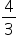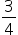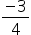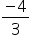Hint:

## The correct answer is:### The given number is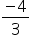.A multiplicative inverse of a number is a number when multiplied with given number gives the value 1. It is the reciprocal of the given number.Let the multiplicative inverse of the given number be x.So, -× x = 1Dividing both the sides by -3/4 we getx =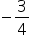So, the multiplicative inverse of the given number is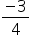.

We just need to find the reciprocal of the given number to find a multiplicative inverse.#### With Turito Foundation.#### Get an Expert Advice From Turito.